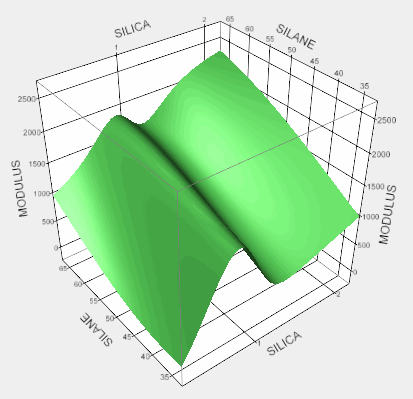Profilers > Surface Plot
Publication date: 08/13/2020

# Surface Plot

##### Explore Contours of Responses across Three Factors

A surface plot is a three-dimensional plot with one or more dependent variables represented by a smooth surface. You can display up to four dependent variables on the same surface plot.

JMP produces surface plots in the following two situations:

The Surface Plot platform creates a stand-alone report that contains a surface plot for formulas. The formulas can be formula columns in your data table or mathematical formulas that do not involve any data points.

The Surface Profiler option in many model fitting platforms produces a surface plot for the fitted model in the existing platform report.

Figure 5.1 Example of a Surface Plot